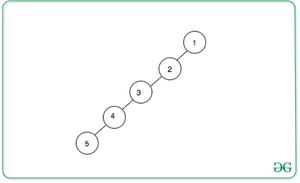Check if a graph constructed from an array based on given conditions consists of a cycle or not

• Difficulty Level : Medium
• Last Updated : 22 Jun, 2021

Given an array arr[] consisting of first N natural numbers, construct an undirected graph using the array elements such that for any array element, connect an edge with the next greater element on the left as well as right.

Examples:

Input: arr = {1, 2, 3, 4, 5}
Output: No
Explanation:
It is clear from the below image that final graph will be a tree.Input: arr[] = {1, 4, 2, 5, 3}
Output: Yes

Naive Approach: The simplest approach is to construct the required graph using the above conditions and check if there exists any cycle of at least length 3 or not. If there exists a cycle, then print “Yes“. Otherwise, print “No“.
Time Complexity: O(N + E), where E is the number of edges.
Auxiliary Space: O(N)

Efficient Approach: The optimal idea is to check if the given permutation is unimodal or non-unimodal, i.e.simply check if there exists any array element with greater adjacent elements on both sides. If found to be true, print “Yes”. Otherwise, print “No”.
Below is the implementation of the above approach:

C++

 // C++ program for the above approach #include using namespace std; // Function to check if the graph// constructed from given array// contains a cycle or notvoid isCycleExists(int arr[], int N){    bool valley = 0;     // Traverse the array    for (int i = 1; i < N; i++) {         // If arr[i] is less than        // arr[i - 1] and arr[i]        if (arr[i] < arr[i - 1]            && arr[i] < arr[i + 1]) {             cout << "Yes" << endl;            return;        }    }     cout << "No";} // Driver Codeint main(){    // Given array    int arr[] = { 1, 3, 2, 4, 5 };     // Size of the array    int N = sizeof(arr)            / sizeof(arr);     isCycleExists(arr, N);     return 0;}

Java

 // Java program for the above approachimport java.io.*;class GFG{   // Function to check if the graph  // constructed from given array  // contains a cycle or not  static void isCycleExists(int[] arr, int N)  {     // Traverse the array    for (int i = 1; i < N; i++)    {       // If arr[i] is less than      // arr[i - 1] and arr[i]      if (arr[i] < arr[i - 1]          && arr[i] < arr[i + 1])      {        System.out.println("Yes");        return;      }    }    System.out.println("No");  }   // Driver Code  public static void main(String[] args)  {     // Given array    int[] arr = { 1, 3, 2, 4, 5 };     // Size of the array    int N = arr.length;    isCycleExists(arr, N);  }} // This code is contributed by Dharanendra L V.

Python3

 # Python3 program for the above approach # Function to check if the graph# constructed from given array# contains a cycle or notdef isCycleExists(arr, N):    valley = 0     # Traverse the array    for i in range(1, N):         # If arr[i] is less than        # arr[i - 1] and arr[i]        if (arr[i] < arr[i - 1] and arr[i] < arr[i + 1]):            print("Yes")            return    print("No") # Driver Codeif __name__ == '__main__':       # Given array    arr = [1, 3, 2, 4, 5]     # Size of the array    N = len(arr)    isCycleExists(arr, N) # This code is contributed by mohit kumar 29

C#

 // C# program for the above approachusing System;class GFG{   // Function to check if the graph  // constructed from given array  // contains a cycle or not  static void isCycleExists(int[] arr, int N)  {     // Traverse the array    for (int i = 1; i < N; i++)    {       // If arr[i] is less than      // arr[i - 1] and arr[i]      if (arr[i] < arr[i - 1]          && arr[i] < arr[i + 1])      {        Console.WriteLine("Yes");        return;      }    }    Console.WriteLine("No");  }   // Driver Code  public static void Main()  {         // Given array    int[] arr = { 1, 3, 2, 4, 5 };     // Size of the array    int N = arr.Length;    isCycleExists(arr, N);  }} // This code is contributed by chitranayal.

Javascript


Output:
Yes

Time Complexity: O(N)
Auxiliary Space: O(1)

My Personal Notes arrow_drop_up